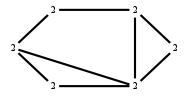The Markov Bases Database

G148_bin

The binary graph model of G148.

It is a hierarchical model of 6 variables. The dimension of the model is 14.The cardinality of the statespace is 64.

Properties of the Markov basis

 Markov degree 4 4917

 degree # of gen. 2 4 328 4589

The model has the following properties:

• All variables are binary.
• It is a graph model.
• The semigroup is normal.

The following properties of the model are unknown:

• The minimal Markov basis may be unique.FILES

 Markov basis: sufficient statistics matrix: G148_bin.mar (926.75 kb) G148_bin.mat (4.04 kb) G148_bin.mod (65 b) G148_bin.tar.gz (36.02 kb)

Wrong or missing information? Write us an email.

page last update on November 15 2020. ->Contact